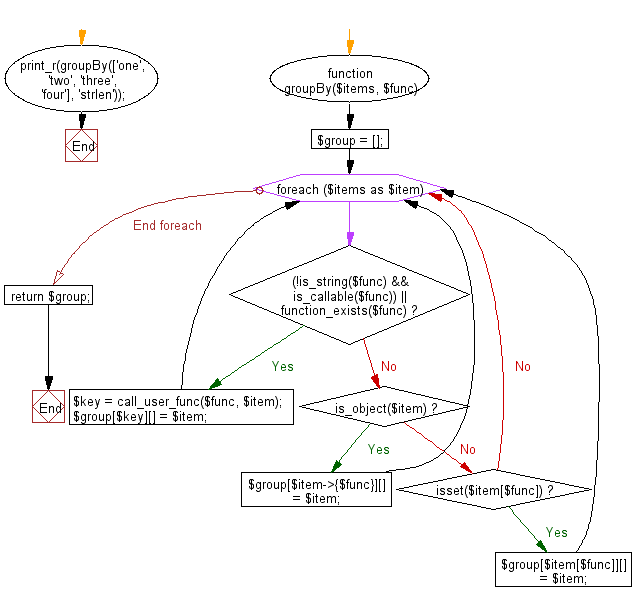﻿ PHP Exercise: Group the elements of an array based on the given function - w3resource

# PHP Exercises: Group the elements of an array based on the given function

## PHP: Exercise-83 with Solution

Write a PHP program to group the elements of an array based on the given function.

Sample Solution: -

PHP Code:

``````<?php
function groupBy(\$items, \$func)
{
\$group = [];
foreach (\$items as \$item) {
if ((!is_string(\$func) && is_callable(\$func)) || function_exists(\$func)) {
\$key = call_user_func(\$func, \$item);
\$group[\$key][] = \$item;
} elseif (is_object(\$item)) {
\$group[\$item->{\$func}][] = \$item;
} elseif (isset(\$item[\$func])) {
\$group[\$item[\$func]][] = \$item;
}
}
return \$group;
}
print_r(groupBy(['one', 'two', 'three', 'four'], 'strlen'));

?>
```
```

Sample Output:

```Array
(
 => Array
(
 => one
 => two
)

 => Array
(
 => three
)

 => Array
(
 => four
)

)```

Flowchart:PHP Code Editor:

Have another way to solve this solution? Contribute your code (and comments) through Disqus.

What is the difficulty level of this exercise?

Test your Programming skills with w3resource's quiz.

﻿